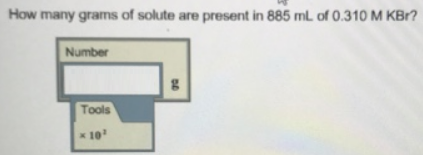Chemistry Practice Problems Molarity Practice Problems Solution: How many grams of solute are present in 885 mL of ...

# Solution: How many grams of solute are present in 885 mL of 0.310 M KBr?

###### Problem

How many grams of solute are present in 885 mL of 0.310 M KBr?View Complete Written Solution

Molarity

Molarity

#### Q. What is the concentration (M) of lithium ions in 4.57 L of a 2.35 M Li  3P solution?a. 45.0 Mb. 14.1 Mc. 2.35 Md. 7.05 Me. 99.0 M

Solved • Tue Jul 31 2018 11:35:30 GMT-0400 (EDT)

Molarity

#### Q. What is the concentration (in M) of methanol in an aqueous solution if dissolving 1.25 mol of methanol in water produces a 10.0 L solution? A. 125 x 1...

Solved • Tue Jul 24 2018 15:46:17 GMT-0400 (EDT)

Molarity

#### Q.  A 365 ml can of soda pop contains 45 g of sucrose (C12H22O11) What is the molarity of the solution with respect to sucrose Express your answer using ...

Solved • Tue Jul 24 2018 09:47:09 GMT-0400 (EDT)

Molarity

#### Q. Calculate the molarity of the two solutions. a) 0.300 mol of NaOH in 1.80 L of solution. b) 14.7 g of NaCI in 719 mL of solution.

Solved • Mon Jul 16 2018 16:16:48 GMT-0400 (EDT)